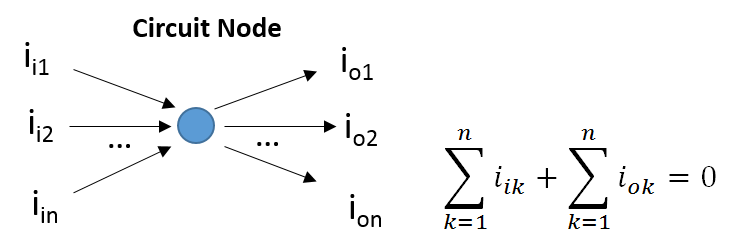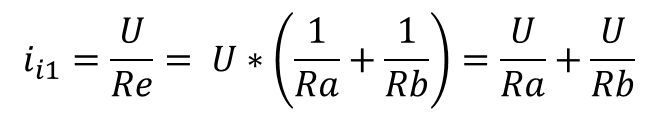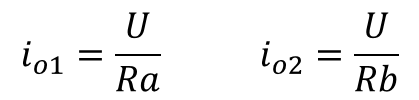# Laws of electronics: KCL

This post describes one of the basic laws of circuit analysis, more precisely KCL (Kirchhoff’s Current Law), which states that the the sum of the currents leaving and entering a node is zero.

KCL

Kirchhoff’s Current Law states that the the sum of the currents leaving and entering a node is zero.

We need to take into account the magnitude and the signal of the current, as we did for KVL. To do so, we define that the currents entering a node are positive and the currents leaving it are negative (or the opposite, as long as we maintain the rule for every current). Figure 1 illustrates the application of the law.Figure 1 – KCL law exemplification.

In figure 2, we present a simple circuit to demonstrate that this law holds true. First, we simplify the circuit applying the rule for parallel resistors.Figure 2 – Application of KCL to a resistive circuit.

From this simplification and using Ohm’s law, we calculate the total amount of current flowing in the circuit:From the circuit before simplification, using again Ohm’s law, we calculate the current flowing in each resistor:Finally, we validate KCL on node A:As stated for KVL, KCL is derived from Maxwell’s equations, which are the fundamental equations for electricity and magnetism.

### 1 thought on “Laws of electronics: KCL”

1.i have pdf on my blog on NETWORK THEORY

2.i have pdf on my blog on NETWORK THEORY